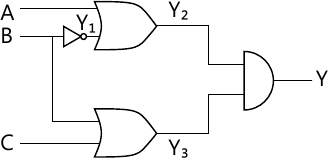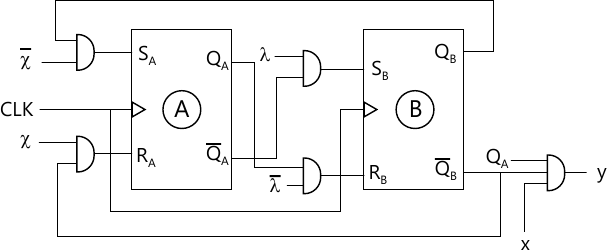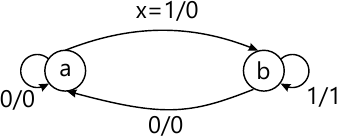MORE IN Design of Programming with Logic
VTU Computer Science (Semester 3)
Design of Programming with Logic
June 2012
Total marks: --
Total time: --
INSTRUCTIONS
(1) Assume appropriate data and state your reasons
(2) Marks are given to the right of every question
(3) Draw neat diagrams wherever necessary

1 (a) Resize basic gates, using only NOR gates.
6 M
1 (b) State the DeMorgan's theorem for two variables and prove the same using perfect induction.
6 M
1 (c) What is HDL? Explain verilog program the structures.
8 M

2 (a) Using K-Map technique simplify
f(a,b,c,d)=∑(1,2,4,5,6,8,9,11,15)+dc(3,7,13).
5 M
2 (b) Using Quine Mc Clusky method simplify,
f(w,x,y,z)=∑=(0,1,3,4,7,12,14,15)
10 M
2 (c) Does circuit in Fig.2(c), experience hazard? If so, verigy the same with timing diagram.5 M

3 (a) Prove that a4:1 Mux can be realized, using only 2:1 multiplexers.
6 M
3 (b) Using a 3:8 decoder realize a full adder.
6 M
3 (c) Implement the following Boolean functions, using suitable PLA.
f1=∑(0,1,4,6),
f2=∑(2,3,4,6,7)
f3=∑=(0,1,2,6)
f4=∑(2,3,5,6,7)
8 M

4 (a) Explain the characteristics of an ideal clock.
4 M
4 (b) What do you mean by characteristics equation of a flip-flop? Derive characteristics equation for S.R. Flip-Flop.
6 M
4 (c) Write the state table and state diagram for the circuit shown in Fig.Q4(c).10 M

5 (a) With neat timing diagram, explain the working of a 4-bit SISO register.
10 M
5 (b) With neat diagram, explain how 7495 can be connected to function as switched tail counter,
5 M
5 (c) Write verilog code for Johnson counter.
5 M

6 (a) Design mod-12 counter using 7493.
4 M
6 (b) What do you mean by lockout condition in counters? Using J.K Flip-Flops design self correcting mod-6 counter.
12 M
6 (c) Bring out the differences between synchronous and asynchronous counters.
4 M

7 (a) With the aid neat block diagrams, define Mealy and Moore machines.
6 M
7 (b) Draw the ASM chart for Mealy machine shown in Fig. Q7(b).4 M
7 (c) Reduce the state table in Table Q7(c),using implication table method.
 PS NS O/P x=0 x=1 x=0 x=1 a h c 1 0 b c d 0 1 c h b 0 0 d f h 0 0 e c f 0 1 f f g 0 0 g g c 1 0 h a c 1 0
10 M

8 (a) With neat circuit diagram, explain the working of R-2R ladder DAC.
8 M
8 (b) Explain the working of ADC.
8 M
8 (c) Calculate conversion time for 10-bit ADC operating at 5MHz clock.
4 M

More question papers from Design of Programming with Logic